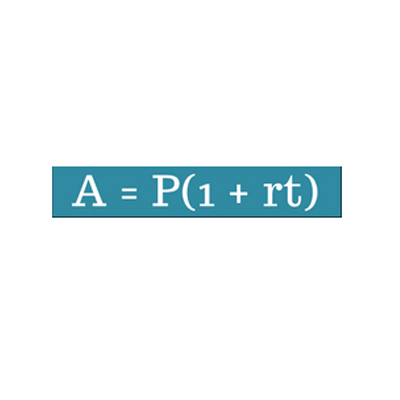# Simple Interest Calculator

Simple interest is money you can earn by initially investing the principal amount. A percentage i.e. the interest of the principal is added to the principal. The Interest is the fee charged for borrowing money. Think of this fee as rent charged for using someone else money. Interest rates are a computed percentage of the principal over a certain amount of time. Interest rates are usually annual.

The Principal is a sum of money that you borrow from a lending institution, or deposit in a savings account.

Enter the Principal Amount (P), Rate of Interest (R) per Year and Time Period (t) term per Year to calculate the simple interest.## The Simple Interest Formula

### I=Prt

I : interest

P : principal amount

r : annual simple interest rate

t : time in years

#### We can expand on the simple interest formula we just covered.

If we wanted to calculate how much we would owe someone who lent us money at the end of a time period, including the principal amount and the interest, we can use the formula:

### A=P + Prt

then factor out a P to get:

#### A=P(1+rt)

This is called the Amount: Simple Interest Formula.

The variables P, r and t are the same as they were in the simple interest formula, where:
P=the principal or present value (the amount borrowed)
r=the annual simple interest rate (in decimal form)
t=the time (in years)
A stands for the future value, or the amount a borrower would owe a lender after t years.

### Spreading Knowledge Across the World

USA - United States of America  Canada  United Kingdom  Australia  New Zealand  South America  Brazil  Portugal  Netherland  South Africa  Ethiopia  Zambia  Singapore  Malaysia  India  China  UAE - Saudi Arabia  Qatar  Oman  Kuwait  Bahrain  Dubai  Israil  England  Scotland  Norway  Ireland  Denmark  France  Spain  Poland  and  many more....